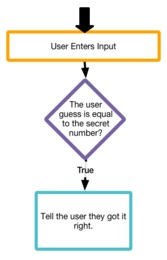# If statement

Category:Control

## if statement

Category: Control

Executes a block of statements if the specified condition is true.

Apps usually need to make decisions and execute some code if something is true. Most programming languages have an if statement: to check to see if some expression is true, and if it is do something.

An if statement requires you to define an expression that evaluates to true or false. Just as in arithmetic there are some operators you can use to write expressions that evaluate to a number, programming languages also have a comparison operators (< <= == > >= !=) and boolean operators (&& || !) that let you write expressions that evaluate to true or false.

The if statement defines a block of code to execute between open and closing curly braces {}. If the condition is true then the block of code inside the curly braces is executed from top to bottom, exactly once. If the condition is false then the entire block of code is ignored.

### Examples

```// Prompts the user for the number of hours they worked and tells them if they worked overtime.
var hoursWorked = promptNum("How many hours did you work this week?");
if (hoursWorked > 40) {
write("You worked " + (hoursWorked-40) + " hours overtime.");
}
```

Example: Guess My Number Prompts the user to guess a secret number between 1 and 10.

 ```// Prompts the user to guess a secret number between 1 and 10. var secretNumber=randomNumber(1,10); var guess = promptNum("Guess my number from 1 to 10."); if (secretNumber == guess) { write("You guessed it!"); } write("Thanks for playing."); ```Example: Time Waster Five clicks and you are done.

```// Five clicks and you are done.
var count = 0;
button("id", "Click Me!");
onEvent("id", "click", function(event) {
count = count + 1;
if (count == 5) {
deleteElement("id");
write("Five clicks and you are done.");
}
});
```

### Syntax

```if (condition) {
statement
}
```

### Parameters

Name Type Required? Description
condition boolean expression Yes An expression that evaluates to true or false. Comparison operators include < <= == > >= !=. Boolean operators include &&
statement App Lab statement(s) Yes Any valid App Lab statements.

No return value.

### Tips

• Unlike an event handler, an if statement does not constantly monitor your program checking the condition to see if it's true or false. An if statement is an instruction just like any other that gets executed line by line in order from top to bottom.
• = is the assignment operator. == is the boolean check for equivalency operator.

Found a bug in the documentation? Let us know at documentation@code.org

## Syntax

```if(  ){ //code }
```

Found a bug in the documentation? Let us know at documentation@code.org